# Problem Solving Shortcuts through the Problem Space Problem

• Slides: 13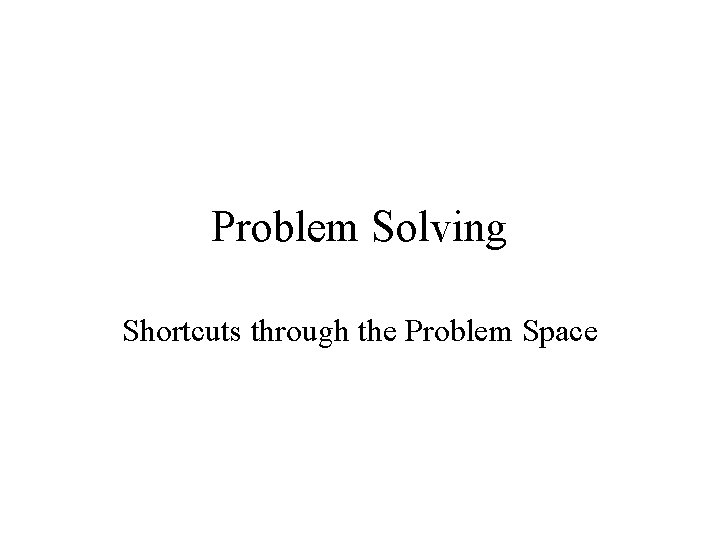Problem Solving Shortcuts through the Problem SpaceProblem Solving • Problem = a situation in which one is trying to reach a goal • Problem solving = finding a means for arriving at a goal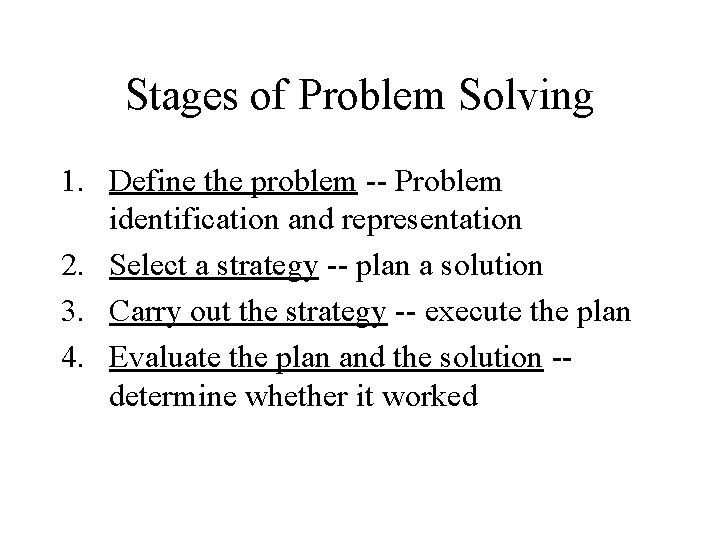Stages of Problem Solving 1. Define the problem -- Problem identification and representation 2. Select a strategy -- plan a solution 3. Carry out the strategy -- execute the plan 4. Evaluate the plan and the solution -determine whether it worked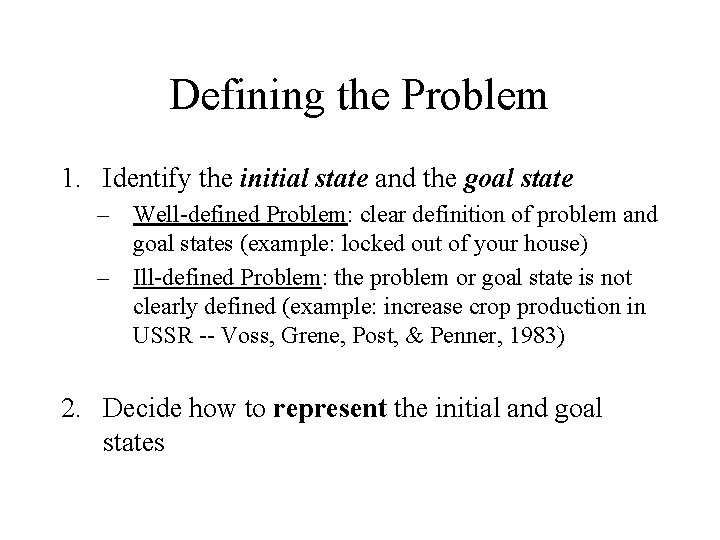Defining the Problem 1. Identify the initial state and the goal state – Well-defined Problem: clear definition of problem and goal states (example: locked out of your house) – Ill-defined Problem: the problem or goal state is not clearly defined (example: increase crop production in USSR -- Voss, Grene, Post, & Penner, 1983) 2. Decide how to represent the initial and goal statesProblem Representation • How difficult it is to solve a problem often depends on how you choose to represent it. • Some examples: – "number scrabble" (Newell & Simon, 1972) – "Bird and Trains" math problem (from Posner 1973, Cognition: An introduction) – The monk problem (Duncker, 1945)Selecting a Strategy: Algorithms vs. Heuristics • Algorithm – a procedure that is guaranteed to produce a solution to the problem – Examples: • solving the anagram "xbo“ by enumerating all possible combinations: xbo, xob, oxb, obx, bxo, box • What about "ntraoc"? There are 6! (or 72) possible combinations.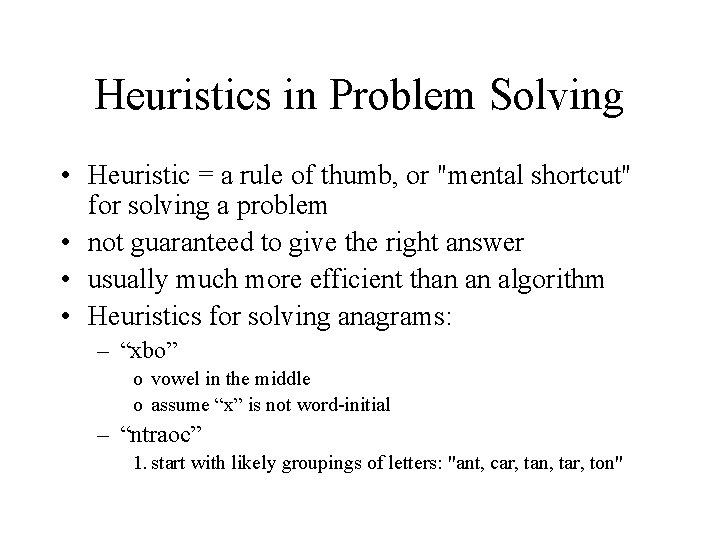Heuristics in Problem Solving • Heuristic = a rule of thumb, or "mental shortcut" for solving a problem • not guaranteed to give the right answer • usually much more efficient than an algorithm • Heuristics for solving anagrams: – “xbo” o vowel in the middle o assume “x” is not word-initial – “ntraoc” 1. start with likely groupings of letters: "ant, car, tan, tar, ton"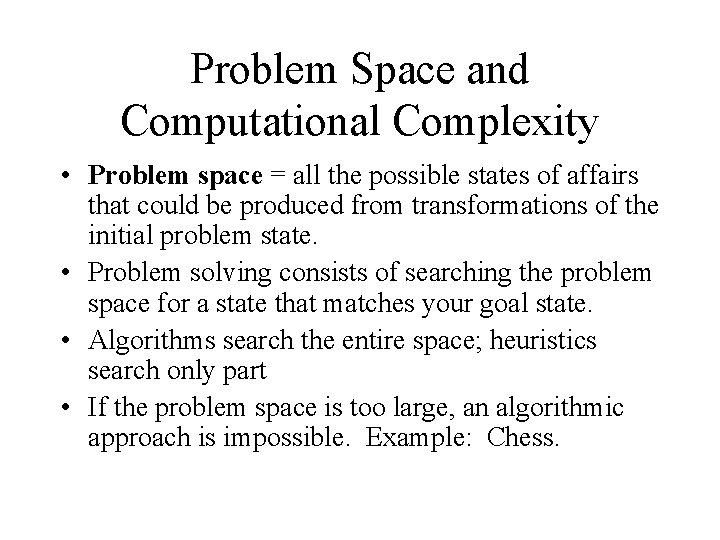Problem Space and Computational Complexity • Problem space = all the possible states of affairs that could be produced from transformations of the initial problem state. • Problem solving consists of searching the problem space for a state that matches your goal state. • Algorithms search the entire space; heuristics search only part • If the problem space is too large, an algorithmic approach is impossible. Example: Chess.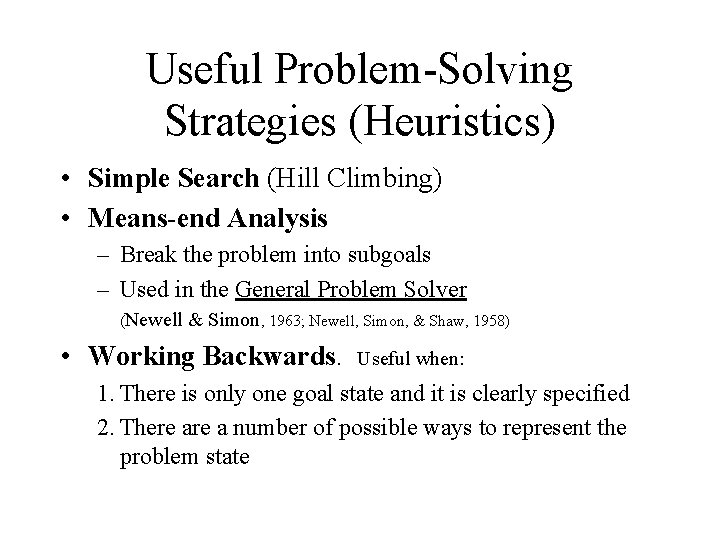Useful Problem-Solving Strategies (Heuristics) • Simple Search (Hill Climbing) • Means-end Analysis – Break the problem into subgoals – Used in the General Problem Solver (Newell & Simon, 1963; Newell, Simon, & Shaw, 1958) • Working Backwards. Useful when: 1. There is only one goal state and it is clearly specified 2. There a number of possible ways to represent the problem state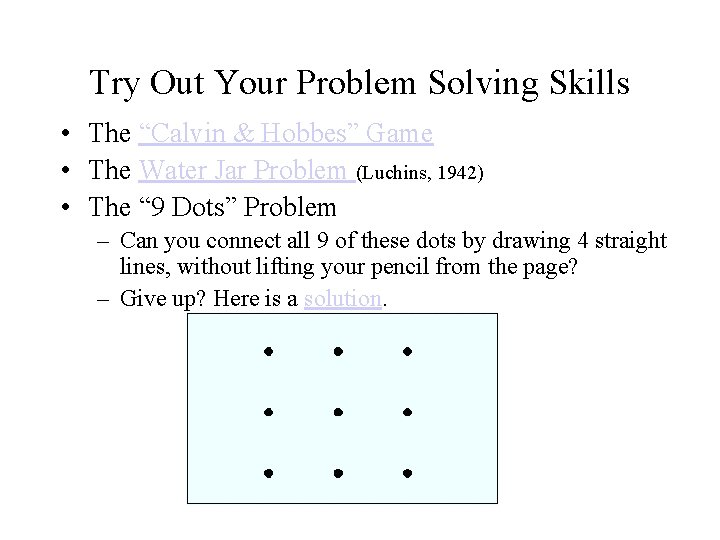Try Out Your Problem Solving Skills • The “Calvin & Hobbes” Game • The Water Jar Problem (Luchins, 1942) • The “ 9 Dots” Problem – Can you connect all 9 of these dots by drawing 4 straight lines, without lifting your pencil from the page? – Give up? Here is a solution.“Set” in Problem Solving • "set" = state of mind a person brings to a problem solving situation • An inappropriate "set" can keep you from representing the problem in the most productive way, or from choosing the best solution strategy.“Set” interfering with problem representation • • The nine dots problem -- including an unnecessary boundary in your representation of the problem Functional Fixedness: failing to see a new use for an object – Duncker (1945) -- mount a candle on the wall – The two string problem“Set” interfering with choosing an effective solution strategy • "Persistence of set" in the water jar problem (Luchins, 1942)